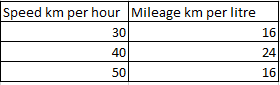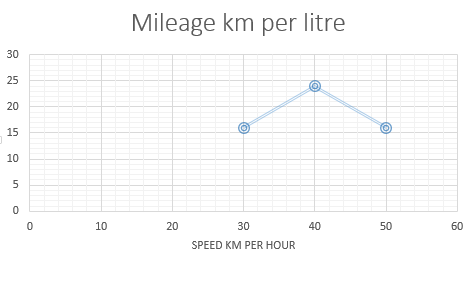### CAT 1999 Question Paper Question 51

Instructions

DIRECTIONS for the following questions: These questions are based on the situation given below: Rajiv reaches city B from city A in 4 hours, driving at the speed of 35 km per hour for the first 2 hours and at 45 km per hour for the next two hours. Aditi follows the same route, but drives at three different speeds: 30, 40 and 50 km per hour, covering an equal distance in each speed segment. The two cars are similar with petrol consumption characteristics (km per litre) shown in the figure below.Question 51

# The amount of petrol consumed by Aditi for the journey is

Solution

Total distance = 35*2 + 45*2 = 160 km

Distance traveled by Aditi at each constant speed value = $$\frac{160}{3}$$

Total petrol consumed by Aditi = $$\frac{160/3}{16}$$ + $$\frac{160/3}{24}$$ + $$\frac{160/3}{16}$$ = $$\frac{10}{3}$$ + $$\frac{20}{9}$$ + $$\frac{10}{3}$$ = 6.67 + 2.22 = 8.9 litres